# Intersection tools

The intersection tools are interactive construction tools that solve basic analytic geometry problems such as finding the intersection of two distances or the midpoint between two coordinate locations. Intersection tools are available on the construction toolbar and work with the active feature construction tool to create vertices or point features at specific locations.

The following diagram illustrates the type of geometric relationships these tools can solve using specified measurements and two known origin locations shown as points A and B:

##### Note:

When ground to grid correction is turned on, your input values are assumed to be ground measurements. An offset angle is applied to directions, and a distance factor multiplies distances. This occurs in real time as you create geometry using construction tools and type bearing and distance values.

ToolDescription

Distance Distance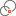Create a point or vertex at the intersection of two distances

Direction Distance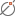Create a point or vertex at the intersection of a direction and distance

Direction Direction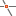Create a point or vertex at the intersection of two directions

Intersection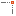Create a point or vertex at an inferred intersection

Midpoint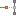Create a point or vertex at the midpoint of two locations